## Cordic in MATLAB

Let’s z be a 2D point in the space as $z = x + jy$, if we want to rotate this point a given angle $\theta$, we get the following expressions:
$e^{j\theta} \cdot z = \left(\cos{\theta} + j \sin{\theta}\right)\left(x+jy\right) \\ = x\cos{\theta}-y\sin{\theta} + j \left(y \cos{\theta} + x \sin{\theta} \right) \\ = x’ + j y’$

Then, for a generic point, the rotation can be expressed as an equation system, where $x’$ and $y’$ are the new coordinates, $\theta$ is the rotation angle and $x$ and $y$ are the original coordinates:
$\begin{bmatrix} x’\\ y’ \end{bmatrix}= \begin{bmatrix} \cos{\theta} & -\sin{\theta}\\ \sin{\theta} & \cos{\theta} \end{bmatrix}\begin{bmatrix} x\\ y \end{bmatrix}$

This rotation can be coded in MATLAB as:

A possible implementation of the cordic algorithm could be:

I have coded an interactive applet to illustrate the algorithm. It has been done using the p5.js library. The error limit has been set to $0.5$.

## Install Quartus in Ubuntu 16.04

1. Open http://dl.altera.com/?edition=lite
2. Login and click on desired Quartus version download
3. Click in the individual file links to start download (Akamai DLM3 Download Manager might not work).
4. Extract Quartus installer.
5. Run setup.sh: ./setup.sh
6. Select desired devices.
7. For launching modelsim, install libxft2 32 bit version library: sudo apt install libxft2:i386. Then execute ./vsim in the path intelFPGA_lite/17.1/modelsim_ase/linuxaloem/

## Rounding in C

Example:

The console output is:

## Phases in UVM

UVM introduces the concept of phases to ensure that all objects are properly configured and connected before starting the runtime simulation. Phases contribute to a better synchronised simulation and enable to the verification engineer to get better modularity of the testbench.

UVM phases consists of:

1. build
2. connect
3. end_of_elaboration
4. start_of_simulation
5. run
1. reset
2. configure
3. main
4. shutdown
6. extract
7. check
8. report
9. final

The run phase has been simplified to get a better picture of how phases worked. Nevertheless, all subphases in the run phase have a pre_ and post_ phase to add flexibility. Therefore, the run phase is actually composed by the following phases:

1. run
1. pre_reset
2. reset
3. post_reset
4. pre_configure
5. configure
6. post_configure
7. pre_main
8. main
9. post_main
10. pre_shutdown
11. shutdown
12. post_shutdown

Although all phases play an important role, the most relevant phases are:

• build_phase: objects are created
• connect_phase: interconnection between objects are hooked
• run_phase: the test starts. The run_phase is the only phase which is a task instead of a function, and therefore is the only one that can consume time in the simulation.

UVM phases are executed from a hierarchical point of view from top to down fashion. This means that the first object that executes a phase is the top object, usually

testbench  test  environment agent {monitor, driver, sequencer, etc}

Nevertheless, in the connect phase, this happens the other way round in a down to top fashion.

{monitor, driver, sequencer} agent environment test testbench## How to add UVM in your Verilog test bench

To use UVM in your Verilog test bench, you need to compile the UVM package top. To do so, you need to include it on your file by using:

The uvm_pkg is contained in the uvm_pkg.sv that must be passed to the compiler. Therefore, it is necessary to indicate the UVM path to the compiler. In Cadence Incisive Enterprise Simulator (IES) is as easy as to specify -uvm switch.

In Modelsim, from Modelsim console, run:

After compilation, click on Simulate > Start simulation and select the tb in the work library. Then, run the simulation for the desired time.

## Operation with different size operands in Verilog/SystemVerilog

When an operation such as an addtion or a substraction is done using different size operands than final variable, it is necessary to extend sign to ensure the operation is done properly.

#### Wrong:

Sign on data_in will not be respected. data_in will be filled with 0 before doing the operation and won’t be taken as negative (if applies).

#### Correct:

Extend sign to match number of acc_add bits before doing operation

## Instalar minted en Windows 10

minted es un paquete de LaTeX que permite añadir código a tu documento y mostrarlo resaltado. Minted utiliza Python para compilar, por lo que el primer paso es instalar Python 2.6 o superior.

1. Instalar Python
2. Añadir la ruta de Python a las variables del entorno de Windows.
1. Botón derecho en el icono de Windows.
2. Sistema
3. En el buscador añadir «variables» y clickar en «Editar variables del sistema».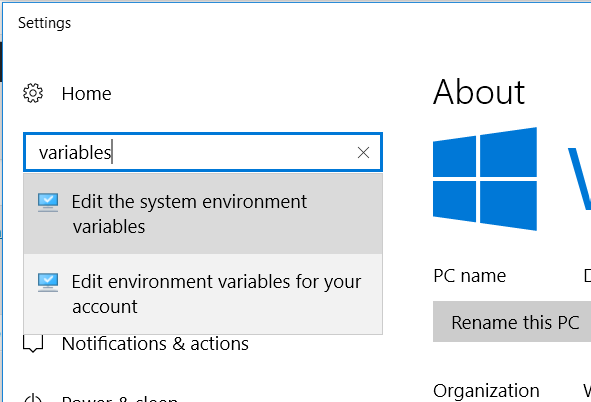4. Clickar en Variables del entorno.
5. En Variables del sistema, añadir a la entrada Path la localización de la carpeta Scripts de Python. En mi caso está en D:\Windows\Python\Python36-32\Scripts. Es muy probable que este paso no sea necesario en tu sistema y que al instalar Python ya se haya añadido la ruta a las variables del sistema. Si al ejecutar python en CMD da un error, entonces este paso es obligatorio.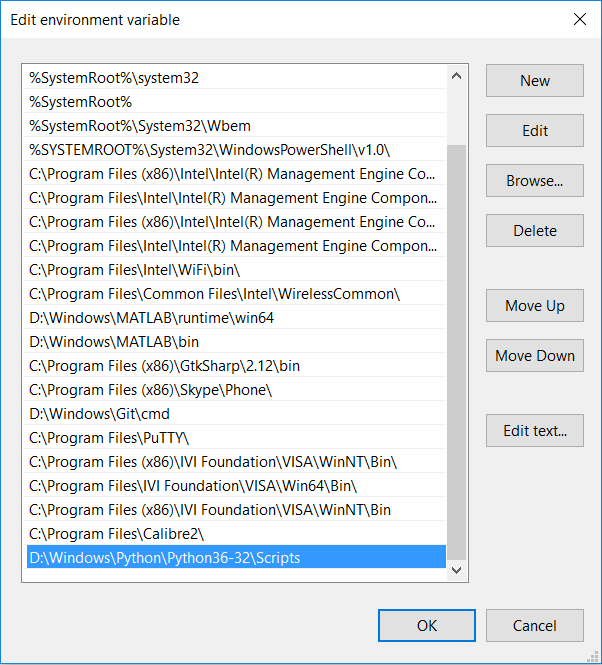6. Instalar pip.
7. Instalar pygments:
1. Abrir CMD en Windows y escribir: pip install Pygments
2. Probar que se ha instalado correctamente escribiendo en CMD pygmentize -h
8. Ahora desde TexStudio hay que modificar la llamada al compilador de LaTeX.
1. Opciones > Configurar TeXStudio > Órdenes
2. Modificar las entradas LaTeX y PdfLaTeX por: latex.exe -src -interaction=nonstopmode -shell-escape %.tex y pdflatex.exe -synctex=1 -interaction=nonstopmode -shell-escape %.tex respectivamente. De esta manera se añade la etiqueta -shell-escape después de -interaction=nonstopmode
3. Cerrar TeXStudio
4. Abrir de nuevo

Y si no ha habido ningún problema en los pasos anteriores, se debería poder compilar utilizando minted. Para más información, visitad la guía de instalación de minted.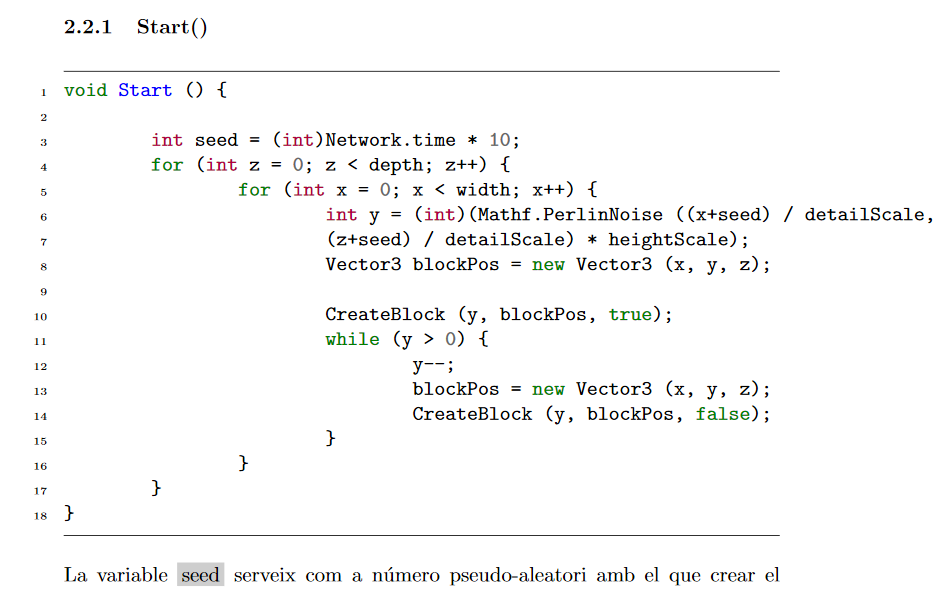## Parameter sweep en Orcad Capture PSpice

1. Instanciar PARAMETERS de la librería SPECIAL.
2. Editar propiedades de PARAMETERSS (doble click).
3. Click en «New property».
4. Añadir parámetro. Ejemplo: Name: Vin_variable, Value: 0.7
5. Modificar valor del componente asignando el nuevo parámetro entre {}. Ejemplo: VAMPL: {Vin_variable}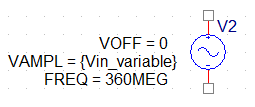6. Añadir «Parametric sweep» en la configuración del perfil de simulación.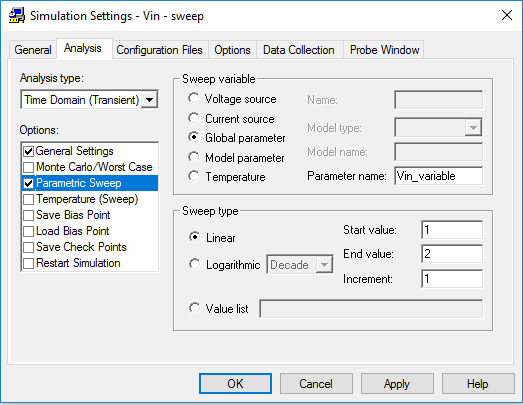7. Por último, realizar la simulación.## Memoria M9K en Cyclone IV

El dispositivo Cyclone IV de Altera dispone de unas memorias embebidas llamadas M9K. Estas memorias son configuradas por el sintetizador (Quartus) y dependiendo del tamaño necesario, se pueden combinar varias. Estas memorias siempre son síncronas, por lo que para que el sintenizador utilice una memoria M9K a partir del código, la memoria en cuestión debe estar gobernada por la señal de reloj.

Estas memorias pueden funcionar con un puerto puerto simple (entrada-salida) o un puerto doble. La configuración de puerto doble consiste en leer de manera independiente una misma memoria por dos puertos. Otra de los modos de funcionamiento es ROM, en el que se escribe el contenido al programar la FPGA y solo es posible leer su contenido.

Dependiendo de la configuración, la capacidad disponible las memorias M9K puede variar. Para anchos de palabra de 1, 2 y 4 bits la capacidad disponible es de 8k (8192 bits). Para tamaños de palabra mayores (8+1), (16+2), (32+4), la capacidad disponible es de 9k (9216 bits).

Las configuraciones en modo ROM son:

• 8k x 1 bit
• 4k x 2 bits
• 2k x 4 bits
• 1k x (8+1) bits
• 512 x (16+2) bits
• 256 x (32+4) bits

Las configuraciones en modo puerto doble son:

• 8k x 1 bit
• 4k x 2 bits
• 2k x 4 bits
• 1k x (8+1) bits
• 512 x (16+2) bits

En el caso de los anchos de palabra no potencias de 2, se puede utilizar estos bits extras para control de errores.

### Ejemplo de memoria 1k x 16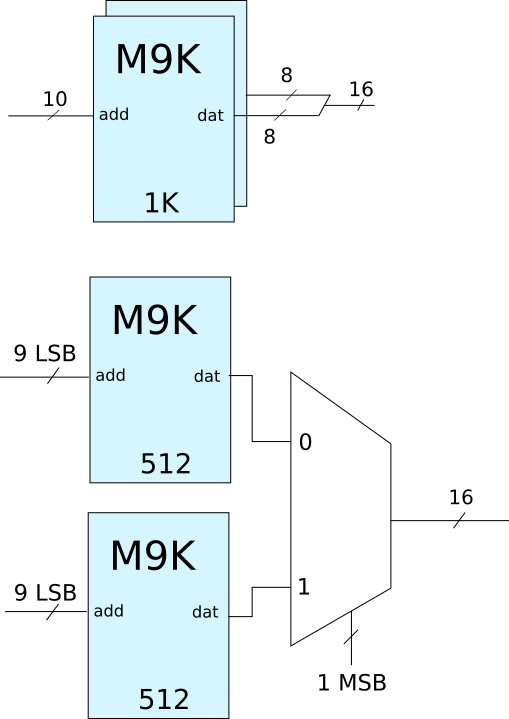La primera opción está más optimizada ya que se necesita menos hardware para obtener el mismo resultado.